Cyclotron

﻿
Cyclotron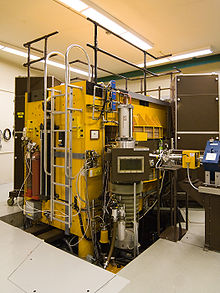A modern Cyclotron for radiation therapy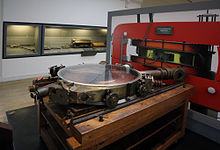A French cyclotron, produced in Zurich, Switzerland in 1937

In technology, a cyclotron is a type of particle accelerator. In physics, the cyclotron frequency or gyrofrequency is the frequency of a charged particle moving perpendicularly to the direction of a uniform magnetic field, i.e. a magnetic field of constant magnitude and direction. Since that motion is always circular, the cyclotron frequency is well defined.

Cyclotrons accelerate charged particles using a high-frequency, alternating voltage (potential difference). A perpendicular magnetic field causes the particles to spiral almost in a circle so that they re-encounter the accelerating voltage many times.

The cyclotron was invented by Leo Szilárd and first manufactured by Ernest Lawrence, of the University of California, Berkeley who started operating it in 1932. Others had been working along similar lines at the time.[citation needed] The first European cyclotron was constructed in Leningrad in the physics department of the Radium Institute, headed by Vitali Khlopin. This instrument was first proposed in 1932 by George Gamow and Lev Mysovskii and was installed and running by 1937.

TRIUMF, Canada's national laboratory for nuclear and particle physics, houses the world's largest cyclotron. The 18m diameter, 4,000 tonne main magnet produces a field of 0.46 T while a 23 MHz 94 kV electric field is used to accelerate the 300 μA beam. TRIUMF is run by a consortium of sixteen Canadian universities and is located at the University of British Columbia, Vancouver, Canada.

How the cyclotron works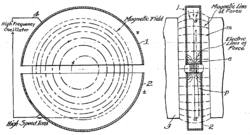Diagram of cyclotron operation from Lawrence's 1934 patent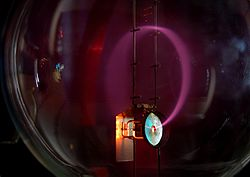Beam of electrons moving in a circle. Lighting is caused by excitation of gas atoms in a bulb.

The electrodes shown at the right would be in the vacuum chamber, which is flat, in a narrow gap between the two poles of a large magnet. In the cyclotron, a high-frequency alternating voltage applied across the "D" electrodes (also called "dees") alternately attracts and repels charged particles. The particles, injected near the center of the magnetic field, increase in speed (and therefore energy) only when passing through the gap between the electrodes. The perpendicular magnetic field (passing vertically through the "D" electrodes), combined with the increasing energy of the particles forces the particles to travel in a spiral path. With no change in energy the charged particles in a magnetic field will follow a circular path. In the cyclotron, energy is applied to the particles as they cross the gap between the dees and so they are accelerated (at the typical sub-relativistic speeds used) and will increase in mass as they approach the speed of light. Either of these effects (increased velocity or increased mass) will increase the radius of the circle and so the path will be a spiral. (The particles move in a spiral, because a current of electrons or ions, flowing perpendicular to a magnetic field, experiences a force perpendicular to its direction of motion. The charged particles move freely in a vacuum, so the particles follow a spiral path.) The radius will increase until the particles hit a target at the perimeter of the vacuum chamber. Various materials may be used for the target, and the collisions will create secondary particles which may be guided outside of the cyclotron and into instruments for analysis. The results will enable the calculation of various properties, such as the mean spacing between atoms and the creation of various collision products. Subsequent chemical and particle analysis of the target material may give insight into nuclear transmutation of the elements used in the target.

Uses of the cyclotron

For several decades, cyclotrons were the best source of high-energy beams for nuclear physics experiments; several cyclotrons are still in use for this type of research. Cyclotrons can be used to treat cancer. Ion beams from cyclotrons can be used, as in proton therapy, to penetrate the body and kill tumors by radiation damage, while minimizing damage to healthy tissue along their path. Cyclotron beams can be used to bombard other atoms to produce short-lived positron-emitting isotopes suitable for PET imaging.

Problems solved by the cyclotron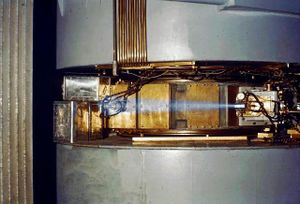60-inch cyclotron, circa 1939, showing a beam of accelerated ions (likely protons or deuterons) escaping the accelerator and ionizing the surrounding air causing a blue glow

The cyclotron was an improvement over the linear accelerators that were available when it was invented. A linear accelerator (also called a linac) accelerates particles in a straight line through an evacuated tube (or series of such tubes placed end to end). A set of electrodes shaped like flat donuts are arranged inside the length of the tube(s). These are driven by high-power radio waves that continuously switch between positive and negative voltage, causing particles traveling along the center of the tube to accelerate. In the 1920s, it was not possible to get high frequency radio waves at high power, so either the accelerating electrodes had to be far apart to accommodate the low frequency or more stages were required to compensate for the low power at each stage. Either way, higher-energy particles required longer accelerators than scientists could afford.

Modern linacs use high power Klystrons and other devices able to impart much more power at higher frequencies. But before these devices existed, cyclotrons were cheaper than linacs. Cyclotrons accelerate particles in a spiral path. Therefore, a compact accelerator can contain much more distance than a linear accelerator, with more opportunities to accelerate the particles.

Advantages of the cyclotron

• Cyclotrons have a single electrical driver, which saves both money and power, since more expense may be allocated to increasing efficiency.
• Cyclotrons produce a continuous stream of particles at the target, so the average power is relatively high.
• The compactness of the device reduces other costs, such as its foundations, radiation shielding, and the enclosing building.

Limitations of the cyclotron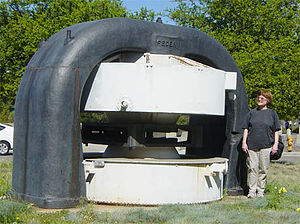The magnet portion of a 27" cyclotron. The gray object is the upper pole piece, routing the magnetic field in two loops through a similar part below. The white canisters held conductive coils to generate the magnetic field. The D electrodes are contained in a vacuum chamber that was inserted in the central field gap.

The spiral path of the cyclotron beam can only "sync up" with klystron-type (constant frequency) voltage sources if the accelerated particles are approximately obeying Newton's Laws of Motion. If the particles become fast enough that relativistic effects become important, the beam gets out of phase with the oscillating electric field, and cannot receive any additional acceleration. The cyclotron is therefore only capable of accelerating particles up to a few percent of the speed of light. To accommodate increased mass the magnetic field may be modified by appropriately shaping the pole pieces as in the isochronous cyclotrons, operating in a pulsed mode and changing the frequency applied to the dees as in the synchrocyclotrons, either of which is limited by the diminishing cost effectiveness of making larger machines. Cost limitations have been overcome by employing the more complex synchrotron or linear accelerator, both of which have the advantage of scalability, offering more power within an improved cost structure as the machines are made larger.

Mathematics of the cyclotron

Non-relativistic

The centripetal force is provided by the transverse magnetic field B, and the force on a particle travelling in a magnetic field (which causes it to be angularly displaced, i.e. spiral) is equal to Bqv. So,$\frac{mv^2}{r} = Bqv$

(Where m is the mass of the particle, q is its charge, B the magnetic field strength, v is its velocity and r is the radius of its path.)

The speed at which the particles enter the cyclotron due to a potential difference, V.$v = \sqrt{\frac{2Vq}{m}}$

Therefore,$\frac{v}{r} = \frac{Bq}{m}$

v/r is equal to angular velocity, ω, so$\omega = \frac{Bq}{m}$

And since the angular frequency is

ω = 2πf

Therefore,$f = \frac{Bq}{2\pi m}$

The frequency of the driving voltage is simply the inverse of this frequency so that the particle crosses between the dees at the same point in the voltage cycle.$f_c = \frac{Bq}{2\pi m}$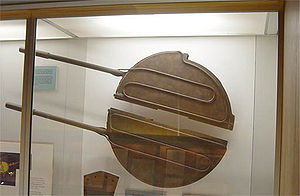A pair of "dee" electrodes with loops of coolant pipes on their surface at the Lawrence Hall of Science. The particle exit point may be seen at the top of the upper dee, where the target would be positioned

This shows that for a particle of constant mass, the frequency does not depend upon the radius of the particle's orbit. As the beam spirals out, its frequency does not decrease, and it must continue to accelerate, as it is travelling more distance in the same time. As particles approach the speed of light, they acquire additional mass, requiring modifications to the frequency, or the magnetic field during the acceleration. This is accomplished in the synchrocyclotron.

Relativistic

The radius of curvature for a particle moving relativistically in a static magnetic field is$r = \frac{\gamma m v}{q B}$
where$\gamma=\frac{1}{\sqrt{1-\left(\frac{v}{c}\right)^2}}$ is the Lorentz factor

Note that in high-energy experiments energy, E, and momentum, p, are used rather than velocity, and both measured in units of energy. In that case one should use the substitution,$\frac{p}{E} = v$
where this is in Natural units

The relativistic cyclotron frequency is$f=f_c\sqrt{1-\left(\frac{v}{c}\right)^2}$,
where
fc is the classical frequency, given above, of a charged particle with velocity
v circling in a magnetic field.

The rest mass of an electron is 511 keV/c2, so the frequency correction is 1% for a magnetic vacuum tube with a 5.11 keV/c2 direct current accelerating voltage. The proton mass is nearly two thousand times the electron mass, so the 1% correction energy is about 9 MeV, which is sufficient to induce nuclear reactions.

An alternative to the synchrocyclotron is the isochronous cyclotron, which has a magnetic field that increases with radius, rather than with time. The de-focusing effect of this radial field gradient is compensated by ridges on the magnet faces which vary the field azimuthally as well. This allows particles to be accelerated continuously, on every period of the radio frequency (RF), rather than in bursts as in most other accelerator types. This principle that alternating field gradients have a net focusing effect is called strong focusing. It was obscurely known theoretically long before it was put into practice.

Related technologies

• The spiraling of electrons in a cylindrical vacuum chamber within a transverse magnetic field is also employed in the magnetron, a device for producing high frequency radio waves (microwaves).
• The Synchrotron moves the particles through a path of constant radius, allowing it to be made as a pipe and so of much larger radius than is practical with the cyclotron and synchrocyclotron. The larger radius allows the use of numerous magnets, each of which imparts angular momentum and so allows particles of higher velocity (mass) to be kept within the bounds of the evacuated pipe. The magnetic field strength of each of the bending magnets is increased as the particles gain energy in order to keep the bending angle constant.

Wikimedia Foundation. 2010.

Look at other dictionaries:

• cyclotron — [ siklɔtrɔ̃ ] n. m. • 1936; de cyclo et (élec)tron ♦ Phys. Accélérateur circulaire de particules (nucléons, ions lourds). Cyclotrons permettant d obtenir des énergies supérieures. ⇒ synchrocyclotron, synchrotron. ● cyclotron nom masculin (de… …   Encyclopédie Universelle

• cyclotron — n. a particle accelerator that imparts energies of several million electron volts to rapidly moving particles; it is used in investigations in nuclear physics and particle physics. [WordNet 1.5 +PJC] …   The Collaborative International Dictionary of English

• cyclotron — 1935, from CYCLO (Cf. cyclo ) + ending from ELECTRON (Cf. electron) …   Etymology dictionary

• cyclotron —  Cyclotron  Циклотрон   Циклический ускоритель нерелятивистских тяжёлых заряженных частиц (протонов, ионов), в котором частицы двигаются в постоянном и однородном магнитном поле, а для их ускорения используется высокочастотное электрическое поле… …   Толковый англо-русский словарь по нанотехнологии. - М.

• cyclotron — ► NOUN ▪ an apparatus in which charged atomic and subatomic particles are accelerated by an alternating electric field while following an outward spiral or circular path in a magnetic field …   English terms dictionary

• cyclotron — ☆ cyclotron [sī′klō trän΄, sī′kləträn΄ ] n. [ CYCLO + TRON] a circular particle accelerator for positively charged ions (usually protons, deuterons, and alpha particles), normally used to initiate nuclear transformations upon collision with a… …   English World dictionary

• Cyclotron — Un aimant du cyclosynchrotron du centre de protonthérapie d Orsay Le cyclotron est un type d’accélérateur de particules circulaire inventé par Ernest Orlando Lawrence en 1931. Dans un cyclotron, les particules placées dans un champ magnétique… …   Wikipédia en Français

• Cyclotron (EP) — Infobox Album | Name = Cyclotron Type = EP Artist = Cyclotron Released = 1997 Recorded = Genre = Industrial metal Length = Label = Allusion Producer = Craig Leach Reviews = * Allmusic Rating|4|5 [http://www.allmusic.com/cg/amg.dll?p=amg… …   Wikipedia

• cyclotron — /suy kleuh tron , sik leuh /, n. Physics. an accelerator in which particles are propelled in spiral paths by the use of a constant magnetic field. [1930 35; CYCLO + TRON] * * * Particle accelerator that accelerates charged atomic or subatomic… …   Universalium

• cyclotron — ciklotronas statusas T sritis automatika atitikmenys: angl. cyclotron vok. Zyklotron, n rus. циклотрон, m pranc. cyclotron, m …   Automatikos terminų žodynas